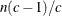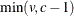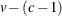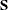# The CANDISC Procedure

### PROC CANDISC Statement

• PROC CANDISC <options>;

The PROC CANDISC statement invokes the CANDISC procedure. Table 31.1 summarizes the options available in the PROC CANDISC statement.

Table 31.1: CANDISC Procedure Options

Option

Description

Input Data Set

Specifies the input SAS data set

Output Data Sets

Specifies the output data set that contains the canonical scores

Specifies the output statistics data set

Method Details

Specifies the number of canonical variables

Specifies a prefix for naming the canonical variables

Specifies the singularity criterion

Control Displayed Output

Displays all output

Displays univariate statistics

Displays between correlations

Displays between covariances

Displays between SSCPs

Displays squared Mahalanobis distances

Suppresses all displayed output

Displays pooled correlations

Displays pooled covariances

Displays pooled SSCPs

Suppresses some displayed output

Displays simple descriptive statistics

Displays standardized class means

Displays total correlations

Displays total covariances

Displays total SSCPs

Displays within correlations

Displays within covariances

Displays within SSCPs

ALL

activates all the display options.

ANOVA

displays univariate statistics for testing the hypothesis that the class means are equal in the population for each variable.

BCORR

displays between-class correlations.

BCOV

displays between-class covariances. The between-class covariance matrix equals the between-class SSCP matrix divided by, where n is the number of observations and c is the number of classes. The between-class covariances should be interpreted in comparison with the total-sample and within-class covariances, not as formal estimates of population parameters.

BSSCP

displays the between-class SSCP matrix.

DATA=SAS-data-set

specifies the data set to be analyzed. The data set can be an ordinary SAS data set or one of several specially structured data sets created by SAS statistical procedures. These specially structured data sets include TYPE=CORR, TYPE=COV, TYPE=CSSCP, and TYPE=SSCP. If you omit the DATA= option, PROC CANDISC uses the most recently created SAS data set.

DISTANCE
MAHALANOBIS

displays squared Mahalanobis distances between the group means, the F statistics, and the corresponding probabilities of greater squared Mahalanobis distances between the group means.

NCAN=n

specifies the number of canonical variables to be computed. The value of n must be less than or equal to the number of variables. If you specify NCAN=0, the procedure displays the canonical correlations but not the canonical coefficients, structures, or means. A negative value suppresses the canonical analysis entirely. Let v be the number of variables in the VAR statement, and let c be the number of classes. If you omit the NCAN= option, onlycanonical variables are generated; if you also specify an OUT= output data set, v canonical variables are generated, and the lastcanonical variables have missing values.

NOPRINT

suppresses the normal display of results. This option temporarily disables the Output Delivery System (ODS). For more information about ODS, see Chapter 20: Using the Output Delivery System.

OUT=SAS-data-set

creates an output SAS data set to contain the original data and the canonical variable scores. If you want to create a SAS data set in a permanent library, you must specify a two-level name. For more information about permanent libraries and SAS data sets, see SAS Language Reference: Concepts.

OUTSTAT=SAS-data-set

creates a TYPE=CORR output SAS data set to contain various statistics, including class means, standard deviations, correlations, canonical correlations, canonical structures, canonical coefficients, and means of canonical variables for each class. If you want to create a SAS data set in a permanent library, you must specify a two-level name. For more information about permanent libraries and SAS data sets, see SAS Language Reference: Concepts.

PCORR

displays pooled within-class correlations (partial correlations based on the pooled within-class covariances).

PCOV

displays pooled within-class covariances.

PREFIX=name

specifies a prefix for naming the canonical variables. By default the names are `Can1`, `Can2`, `Can3`, and so on. If you specify PREFIX=`Abc`, the components are named `Abc1`, `Abc2`, and so on. The number of characters in the prefix plus the number of digits required to designate the canonical variables should not exceed 32. The prefix is truncated if the combined length exceeds 32.

PSSCP

displays the pooled within-class corrected SSCP matrix.

SHORT

suppresses the display of canonical structures, canonical coefficients, and class means on canonical variables; only tables of canonical correlations and multivariate test statistics are displayed.

SIMPLE

displays simple descriptive statistics for the total sample and within each class.

SINGULAR=p

specifies the criterion for determining the singularity of the total-sample correlation matrix and the pooled within-class covariance matrix, where 0 < p < 1. The default is SINGULAR=1E–8.

Letbe the total-sample correlation matrix. If the R square for predicting a quantitative variable in the VAR statement from the variables that precede it exceeds 1 – p, thenis considered singular. Ifis singular, the probability levels for the multivariate test statistics and canonical correlations are adjusted for the number of variables whose R square exceeds 1 – p.

Ifis considered singular and the inverse of(squared Mahalanobis distances) is required, a quasi inverse is used instead. For more information, see the section Quasi-inverse in Chapter 35: The DISCRIM Procedure.

STDMEAN

displays total-sample and pooled within-class standardized class means.

TCORR

displays total-sample correlations.

TCOV

displays total-sample covariances.

TSSCP

displays the total-sample corrected SSCP matrix.

WCORR

displays within-class correlations for each class level.

WCOV

displays within-class covariances for each class level.

WSSCP

displays the within-class corrected SSCP matrix for each class level.